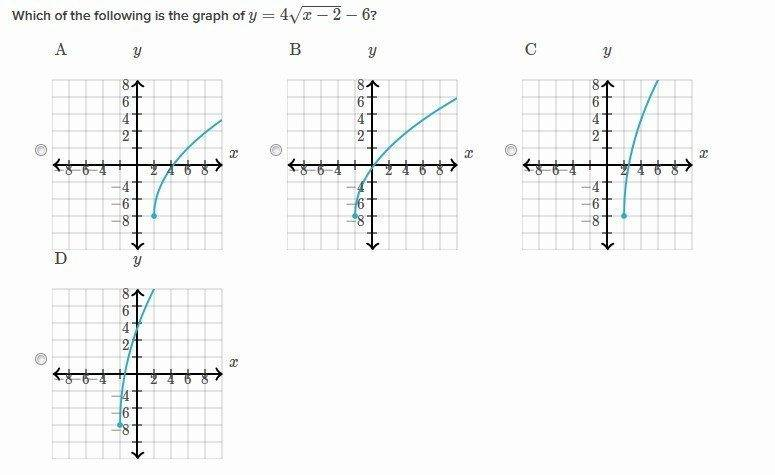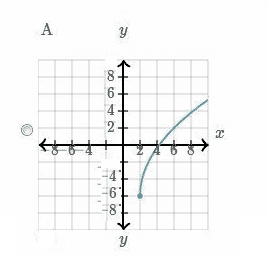# Graphing equation

## Homework Statement

So I have to do one simple task to identify graph to corresponding formula. I dont know if software is wrong or my approach.

y =4√x-2 -6[/B]

## The Attempt at a Solution

Found zero at x = 2 and when x=6 then y=2 and graph is not showing that. Something I missed? Look at picture. The correct answer is A.RUber
Homework Helper
Just to be sure, you are looking at ##4\sqrt{x-2}-6##?
That does not have a zero at x=2.

-6 is not in the sqrt

ehild
Homework Helper

## Homework Statement

So I have to do one simple task to identify graph to corresponding formula. I dont know if software is wrong or my approach.

y =4√x-2 -6[/B]

## The Attempt at a Solution

Found zero at x = 2

No, that is wrong. Solve the equation ##0=4\sqrt{x-2}-6##
and when x=6 then y=2 and graph is not showing that. Something I missed? Look at picture. The correct answer is A.
View attachment 95172

On the y axis, the numbers are above the scales. So the first scale is 2. y=2 at x=6 in figure A.

RUber
Homework Helper
So the zero should be around 4.25, right?

Ok I got it. It was mine and graphs fault.

HallsofIvy
Homework Helper
Rather than solve an equation I would just notice that f(0) is not a real number and f(2)= -6, not -8. None of the given graphs is correct.

Rather than solve an equation I would just notice that f(0) is not a real number and f(2)= -6, not -8. None of the given graphs is correct.

I think graph A is correct, the numbers are just placed in a strange way on the y axis.

ehild
Homework Helper
I think graph A is correct, the numbers are just placed in a strange way on the y axis.
Yes, the numbers are shifted one scale. It should look as in the figure.